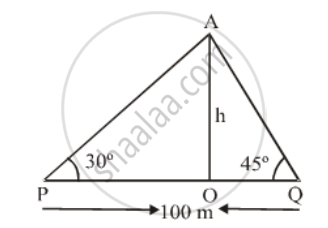# There is a small island in the middle of a 100 m wide river and a tall tree stands on the island. P and Q are points directly opposite to each other on two banks, and in line with the tree. - Mathematics

Sum

There is a small island in the middle of a 100 m wide river and a tall tree stands on the island. P and Q are points directly opposite to each other on two banks, and in line with the tree. If the angles of elevation of the top of the tree from P and Q are respectively 30º and 45º, find the height of the tree

#### Solution

Let OA be the tree of height h metre.

In triangles POA and QOA, we have

\text{tan }30^\text{o}=\frac{OA}{OP}\text{ and tan}45^\text{o}=\frac{OA}{OQ}\Rightarrow \frac{1}{\sqrt{3}}=\frac{h}{OP}\text{ and }1=\frac{h}{OQ}

⇒ OP = √3 h and OQ = h

⇒ OP + OQ = √3 h + h ⇒ PQ = (√3+ 1)h

⇒ 100 = (√3 + 1)h [∵ PQ = 100 m]

\Rightarrow h=\frac{100}{\sqrt{3}+1}\Rightarrowh=\frac{100(\sqrt{3}-1)}{2}\text{ m}

⇒ h = 50(1.732 – 1) m = 36.6 m

Hence, the height of the tree is 36.6 m

Concept: Heights and Distances
Is there an error in this question or solution?